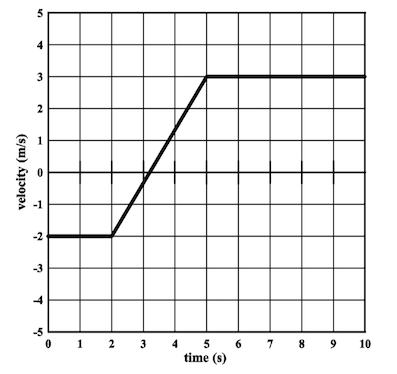Physics Practice Problems Velocity & Acceleration Graphs Practice Problems Solution: A jogger starts from the origin and jogs in a stra...

# Solution: A jogger starts from the origin and jogs in a straight line with the velocity profile shown below. [a] What is the maximum acceleration of the jogger? [b] At what time does the jogger return to the origin? [c] What is the total displacement of the jogger over the time shown?

###### Problem

A jogger starts from the origin and jogs in a straight line with the velocity profile shown below.

[a] What is the maximum acceleration of the jogger?

[c] What is the total displacement of the jogger over the time shown?Velocity & Acceleration Graphs

Velocity & Acceleration Graphs

#### Q. (a) Which graphs represent an object moving in the negative direction?(b) Which graphs represent an object that is speeding up?(c) Which graphs repres...

Solved • Wed Mar 11 2020 11:06:08 GMT-0400 (EDT)

Velocity & Acceleration Graphs

#### Q. A block with an initial velocity v 0 slides up and back down a frictionless incline. Which graph best represents a description of the velocity of the ...

Solved • Wed May 17 2017 12:47:23 GMT-0400 (EDT)

Velocity & Acceleration Graphs

#### Q. Figure 2.2 shows the velocity time graph of a motorist during a 8 second period. The motorist leaves home (position x = 0m) at time t = 0s and the vel...

Solved • Wed Jan 18 2017 14:34:58 GMT-0500 (EST)

Velocity & Acceleration Graphs

#### Q. Figure 2.2 shows the velocity time graph of a motorist during a 8 second period. The motorist leaves home (position x = 0m) at time t = 0s and the vel...

Solved • Wed Jan 18 2017 14:23:30 GMT-0500 (EST)